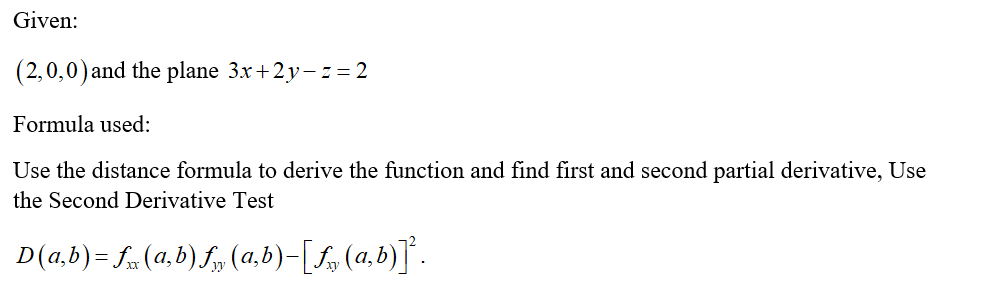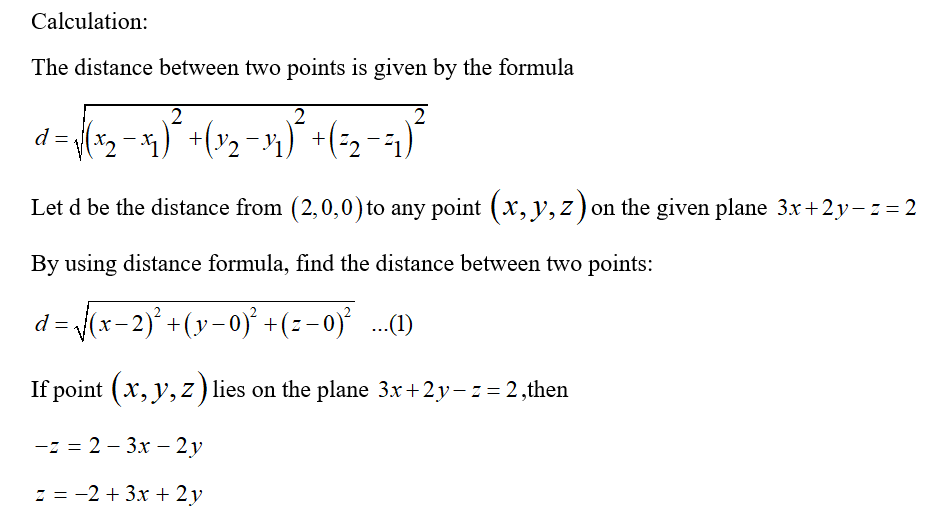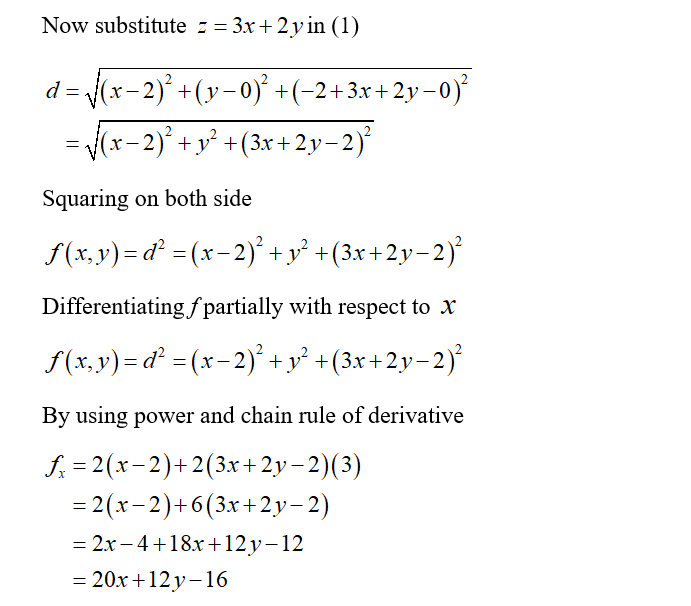# Use partial derivatives to find the coordinates which give the shortest distance from the point (2,0,0) to the plane        3x+2y-z=2. Confirm that the point is the shortest distance with use of second derivatives

Question
1 views

Use partial derivatives to find the coordinates which give the shortest distance from the point (2,0,0) to the plane        3x+2y-z=2. Confirm that the point is the shortest distance with use of second derivatives

check_circle

Step 1Step 2Step 3...

### Want to see the full answer?

See Solution

#### Want to see this answer and more?

Solutions are written by subject experts who are available 24/7. Questions are typically answered within 1 hour.*

See Solution
*Response times may vary by subject and question.
Tagged in

### Calculus